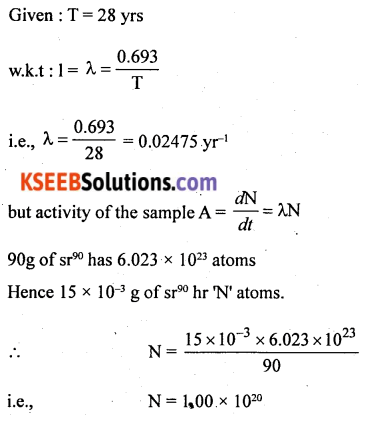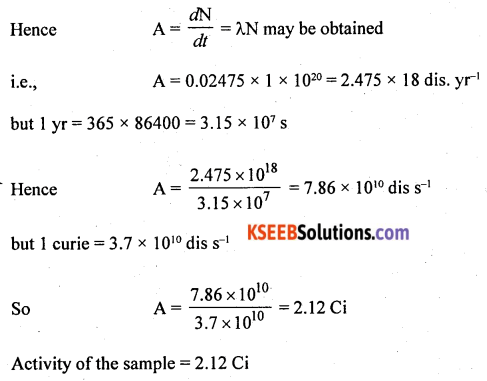# 2nd PUC Physics Previous Year Question Paper June 2018

Students can Download 2nd PUC Physics Previous Year Question Paper June 2018, Karnataka 2nd PUC Physics Model Question Papers with Answers helps you to revise the complete Karnataka State Board Syllabus and score more marks in your examinations.

## Karnataka 2nd PUC Physics Previous Year Question Paper June 2018

Time: 3 hrs 15 min
Max. Marks: 70

Instructions

• All parts are compulsory.
• Answer without relevant diagram/figure/circuit wherever necessary will not carry any marks.
• Numerical problems solved without writing the relevant formulae carry no marks.

Part – A

I. Answer all the following questions: (10 × 1 = 10)

Question 1.
State Ohm’s Law.
Ohm’s law: When all the physical conditions surrounding the conductor remain unaltered, the electric current is directly proportional to the potential difference between its ends.

Question 2.
Define the current sensitivity of a galvanometer.
The number of divisions to which a given MCG gets deflected per one ampere of current in it is known as the current sensitivity of MCG.

Question 3.
Write an expression for force experienced by a straight conductor of length $$\vec{l}$$ carrying a steady current I, moving in a uniform external magnetic field $$\vec{B}$$.
F = BIl sin θ where ‘θ’ is the angle between the magnetic field and length of the conductor.Question 4.
What is ‘retentivity’ in magnetism?
The property of a material to retain the magnetism even after the removal of the magnetizing field is known as retentivity.

Question 5.
Where on the earth’s surface is earth’s surface magnetic dip is zero?
The magnetic dip is zero at the magnetic equator.

Question 6.
State ‘Lenz’s law in electromagnetic induction.
Lenz’s law states that the induced current is always in such a direction as to oppose the cause which produces it.

Question 7.
Write the condition for the ‘resonance’ of the series LCR circuit.
Current through a series LCR circuit will be in phase with the voltage, when inductive reactance (XL) = capacitive reactance (XC). The circuit in this case is said to be under resonance.
Z = Zmin = R; I = Imax

Question 8.
What is ‘wattless’ current?
A component of current that does not allow the circuit elements like capacitors and inductors to dissipate energy in them is called wattless current.Question 9.
A blue ray of light enters an optically denser medium from the air. What happens to its frequency in the denser medium?
The frequency of light remains the same.

Question 10.
$$_{92} \mathrm{U}^{238}$$ undergoes α-decay giving rise to thorium. What is the mass number of the daughter nuclide?Hence the mass number of daughter nucleus = 234

Part – B

II. Answer any FIVE of the following questions. (5 × 2 = 10)

Question 11.
Represent graphically the variation of resistivity with absolute temperature for copper and nichrome metals.
Graphical representation of the variation of resistivity with absolute temperature for copper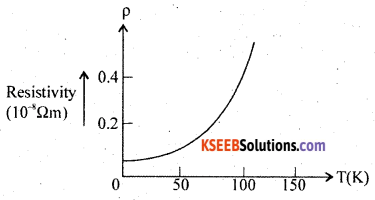Graphical representation of the variation of resistivity with absolute temperature for copper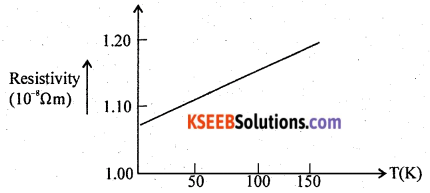Question 12.
Write an expression for cyclotron frequency and explain the terms.
Frequecy ‘f’ = $$\frac{\mathrm{B} q}{2 \pi \mathrm{m}}$$
where B – Magnetic field
q – charge on the particle
m – mass of the perticle

Question 13.
State and explain ‘Curie’s Law’ in magnetism.
The magnetic susceptibility of a paramagnetic substance is inversely proportional to the absolute temperature.Question 14.
Mention any two factors on which the self-inductance of a coil depends.
$$\mathrm{L}=\frac{\mu_{\mathrm{o}} \mu_{\mathrm{r}} \mathrm{N}^{2} \mathrm{A}}{l}$$
Where, N – Number of turns
A – Area of cross section
l – length of a solenoid

Question 15.
Give any two applications of ultraviolet radiation.
(i) Highly focussed UV radiations are used in (LASIK – Laser-Assisted in Situ Keratomileusis)
(ii) UV rays are used in sterilization of air, water, surgical instruments.
The other uses are:
UV rays are used in molecular spectroscopy, identification of artificial gems from real gems.
Note: Ordinary glass absorbs UV radiation.

Question 16.
What is the polarization of light? Name any one method of producing plane polarised light.
The phenomenon of confining the vibrations of the electric vector in a specific direction perpendicular to the direction of wave motion is called polarisation.

• Reflection.

The other methods are by

• refraction
• double refraction
• scattering
• selective absorption.Question 17.
Calculate de Broglie wavelength associated with an electron moving with a speed of 2 × 105 ms-1. Given h = 6.625 × 10-34 Js, me = 9.11 × 10-31 kg.
Given v = 2 × 105 ms-1
h = 6.625 × 10-34 Js
me = 9.11 × 10-31 kgQuestion 18.
Write any two advantages of Light Emitting Diode (LED) over conventional incandescent low power lamps.

• Fast on-off switch ¡ng capabilities.
• From the observed wavelength in the visible range, the wavelengths drift only from 100 Å to 500 Å. Hence the light is nearly monochromatic.
• LEDs consume low power, operate under a very low voltage from 1 .6 V to 2.2 V, and have a long life.

Part – C

III. Answer any five of the following question: (5 × 3 = 15)

Question 19.
Give any three properties of electric field lines.

1. Electric lines of force never intersect with each other.
2. Electric lines of force exert lateral stress on one another.
3. Electric lines of force do not enter a conductor.
4. The direction of the electric field is given by the direction of the tangent drawn at that point on an electric line of force.
5. In the case of a uniform electric field, the electric lines of force remain parallel with each other.
6. Electric flux per unit surface area normal to the flux determines the electric field intensity.

Question 20.
Obtain an expression for the effective capacitance of two capacitors connected in series.
Capacitors are said to be connected in series when

• the same charge appears in each of them
• voltage divides in the inverse ratio of their capacitances.V = V1 + V2 and C = $$\frac{Q}{V}$$ so that V = $$\frac{Q}{C}$$
hence $$\frac{Q}{C}=\frac{Q}{C_{1}}+\frac{Q}{C_{2}}$$
i.e., $$\frac{1}{C}=\frac{1}{C_{1}}+\frac{1}{C_{2}}$$
For ‘n’ unidentical capacitances,
$$\frac{1}{C}=\frac{1}{C_{1}}+\frac{1}{C_{2}}+\frac{1}{C_{3}}+—+\frac{1}{C_{n}}$$
Statement:
The reciprocal of the equivalent capacitance of a number of capacitors connected in series is equal to the algebraic sum of the reciprocals of their individual capacitances.

Note:

• For ‘n’ identical capacitors Cs = $$\frac{C}{n}$$
• For 3 unidenticalcapacitors, $$\frac{1}{C}=\frac{1}{C_{1}}+\frac{1}{C_{2}}+\frac{1}{C_{3}}$$Question 21.
Write any three differences between diamagnetic and paramagnetic materials.
Diamagnetic Substances

1. These substances are repelled by a strong magnetic field.
2. These substances move from a stronger to a weaker magnetic field.
3. 0 ≤ µr < 1
4. ‘χ’ is low but negative and is of the order -10-9 to -10-6.
5. Susceptibility is independent of temperature.

Paramagnetic substances

1. These are slightly attracted to a strong magnetic field.
2. These substances move from a weaker to a stronger magnetic field.
3. 0 ≤ µr < 1 + ∈ where els a small positive integer.
4. ‘χ’ is low but positive and is of the order of +10-6 to +10-4
5. χ ∝ $$\frac{1}{\mathrm{T}}$$
where T is the absolute temperature of the substance.

Question 22.
Describe the coil and bar magnet experiment to demonstrate the phenomenon of electromagnetic induction.
(a) Magnet and coil experiment: If one end of a magnet is suddenly introduced into a coil connected to a sensitive galvanometer it shows a momentary deflection. This shows that a momentary current and hence an instantaneous emf is produced ¡n the circuit and the direction of the current depend on the pole towards the coil. The current produced is called an induced current. If the magnet is withdrawn suddenly, a sudden deflection is again observed but in the opposite direction. The deflection is greater if the magnet is introduced or withdrawn quickly. No induced emf is obtained when there is no relative motion between the coil and the magnet.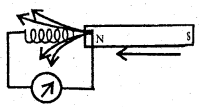(b) Coil and coil experiment: Consider two coils P and S placed closed to each other. Coil P connected to a cell through a key Swiss called the primary coil. The other coil S connected in series with a sensitive galvanometer G is called the secondary coil. On pressing Sw a momentary deflection is noticed in the galvanometer. When the key is released the galvanometer deflects in the opposite direction. However, no induced emf is obtained when a steady current flows through the primary, as long as the secondary coil is at rest.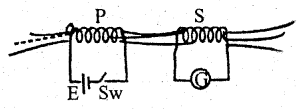Question 23.
Derive an expression for an effective focal length of two thin lenses kept in contact.
(a) Thin lenses in contact:A thin lens is that lens whose thickness can be neglected when compared to its radius of curvature.
By applying a universal lens formula of refraction through L1,
$$\frac{1}{f_{1}}=\frac{1}{-u}+\frac{1}{v^{\prime}}$$ …….. (1)
The image at I’ serves as a virtual object for the thin lens L2. The virtual object distance is taken as +ve in the direction of the incident ray.
$$\frac{1}{f_{2}}=\frac{1}{-v^{\prime}}+\frac{1}{v}$$ …….. (2)
Adding (1) and (2) we get
$$\frac{1}{f_{1}}+\frac{1}{f_{2}}=\frac{1}{-u}+\frac{1}{v}=\frac{1}{f}$$
where f is known as the focal length of equivalent lens.
An equivalent lens is that single lens that produces the same effect as those of combined lenses in contact.
In general,
$$\frac{1}{f}=\frac{1}{f_{1}}+\frac{1}{f_{2}}+\frac{1}{f_{3}}+\ldots \ldots+\frac{1}{f_{n}}$$
Since, P = $$\frac{1}{f}$$ = power of the lens
P = P1 + P2 + P3 + ………. + Pn
P1, P2 may be +ve or -ve depending upon the type of lenses
(b) $$\frac{1}{f}=\frac{1}{f_{1}}+\frac{1}{-f_{2}}$$
where ‘f’ is in metre.
i.e., P = P1 – P2
and ‘P’ is in dioptre.Question 24.
Write any three experimental observations of the photoelectric effect.

• Photoemission is instantaneous
• For every photoemissive surface, there is a minimum frequency of the incident radiation below which there is no photoemission. This minimum frequency is called threshold frequency.
• For frequency greater than the threshold, the strength of the photoelectric current is directly proportional to the intensity of the incident radiation.
• The maximum K.E of the photoelectrons increases linearly with the frequency of the incident radiation.
• There exists a minimum -ve potential called stopping potential for which anode repels the electrons completely.

Question 25.
How Zener diode is used as a voltage regulator? Explain.
Circuit Diagram:The circuit connections are made as shown in the figure. The circuit contains a Zener diode, an unregulated voltage source (Vin > Vz), a series resistance RS, and a load resistance RL. Any increase/decrease of the voltage drop across Rs produces no change in voltage across the Zener diode. For (Vin < Vz), the Zener diode does not function. Thus the Zener diode acts as a voltage regulator.

Question 26.
What is the function of the ‘receiver’ in the communication system? Draw the block diagram of the AM-receiver.
A receiver is a device that detects, demodulates, and amplifies the received message.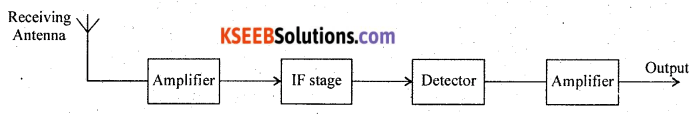Part – D

IV. Answer any two of the following questions: (2 × 5 = 10)

Question 27.
Using Gauss’s law in electrostatics, obtain an expression for the electric field due to a uniformly charged thin spherical shell at a point.
(i) Outside the shell and (ii) Inside the shell
Gauss’s theorem: The total outward electric flux passing through a closed surface in air is ($$\frac{1}{\varepsilon_{0}}$$ ) times the total charge enclosed by it.Let ‘+Q’ be the charge enclosed by a hollow conductor of radius ‘R’.
Let ‘p’ be a point at a distance ‘r’ from the center of the conductor.
Let ‘ds’ be a small element of the area surrounding the point.
A normal drawn from the point coincides with the direction of $$\vec{E}$$.
Hence cos 0° = 1.
From Gauss’ theorem,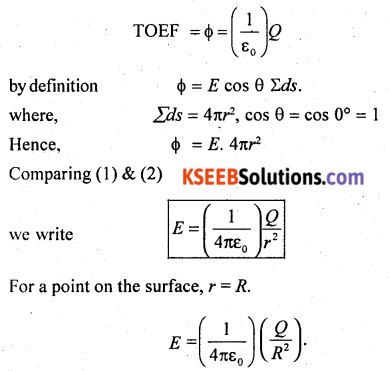This electric field intensity is maximum. Since electric flux depends on the charge enclosed and electric field intensity depends on the electric flux, the electric field remains zero at all points inside the spherical hollow conductor.Question 28.
Derive $$\sigma=\frac{n e^{2} \tau}{m}$$, where the symbols have their usual meaning.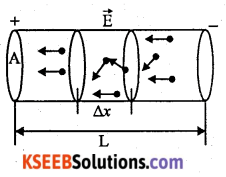Let ‘n’ be the number density of electrons. Let ‘L’ be the length of the conductor, ‘A’ be the area of cross-section of the conductor.
Let vd be the drift velocity of the electrons. Let ∆x be a small length.
Electrons drift in a direction opposite to the electric field.
The number of electrons in ‘∆x’ and area of cross-section ‘A’ is equal to (A∆x)n.
Charge on these electrons = (nA∆x)e
If ∆t is the time taken for effective displacement of electrons then rate of flow charge = nAe($$\frac{\Delta x}{\Delta t}$$)
By definition, electric current I = rate of flow of charge.
i.e., I = nAevd ……. (1)
where the average velocity of electrons with which it drifts against the direction of the electric field is known as drift velocity (vd).
Let ‘a’ be the acceleration of electrons. Let ‘E’ be the electric field intensity. Force on electrons,
F = ma
i.e., eE = ma
but a = vd τ where ‘τ’ is the relaxation time. Relaxation time represents the average time taken for two successive collisions of electrons and ions in the lattice.
Hence, eE = mvd τ or vd = $$=\frac{e E}{m \tau}$$ …….. (2)
We also know that electric potential difference between the ends of the conductor V = EL
$$v_{d}=\frac{e V}{m \tau L}$$ substituting this in the expression (1)
We write, $$I=n A e\left(\frac{e V}{m \tau L}\right)$$
i.e., $$I=\left(\frac{n A e^{2}}{m \tau L}\right) V$$
R = $$\frac{m \tau L}{n A e^{2}}$$ is called the electrical resistance of a conductor.
and K = $$\left(\frac{n A e^{2}}{m \tau L}\right)$$ is called the electric conductance of a conductor.
the expression $$\sigma=\frac{n e^{2}}{m \tau}$$ is called the electrical conductivity and $$\frac{1}{\sigma}=\frac{m \tau}{n e^{2}}$$ is called electrical resistivity.Question 29.
Obtain an expression for the force between two infinitely long straight parallel conductors carrying current. Hence define “ampere” the SI unit of electric current.
X and Y are two long straight parallel conductors carrying currents I1 and I2 respectively and placed close to each other. d is the separation between the two conductors and L is the length of the conductors.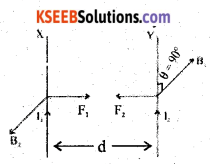The magnetic field at any point on the conductor Y due to current I1 in the conductor X, is given by $$B_{1}=\frac{\mu_{0}}{4 \pi} \frac{2 I_{1}}{d} \cdot \overrightarrow{B_{1}}$$ acts in a direction perpendicular to the plane containing the two conductors. The conductor Y which carries current ‘I2’ experiences a mechanical force due to B1 acting on it and this force is given by F1 = B1 I2 L sin θ.According to Fleming’s left-hand rule, the direction of the force F1 on X is pcrpendicuIarto B2 and is towards the conductor Y.
The magnetic field at any point on the conductor X due to the current I2 in y, is given by $$B_{2}=\frac{\mu_{0}}{4 \pi} \frac{2 I_{2}}{d} \cdot \vec{B}_{2}$$ acts on X and opposite to B1. The mechanical force acts on X due to B2 is I1 L sin θ.According to Fleming’s left-hand side rule, the direct join of the force F2 on X is perpendicular to X and it is towards the conductor y if the current I1 is inwards (or away from the conductor Y if the current I1 is outwards).
The Force F1 acting on a certain length of the conductor Y due to the current in the conductor X is equal in magnitude to the force F2 acting on the same length of X due to the current in conductor Y. If the two conductors carry the currents in the same direction (parallel currents) then the forces attract each other. If the two conductors carry the currents in the opposite directions (anti-parallel currents), then they are found to repel each other because the two forces act away from each other.
The force per unit length on each conductor is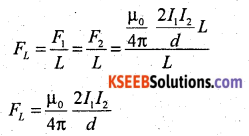One ampere of current can be defined as that constant current which when maintained through each of the two infinitely long straight parallel conductors of the negligible area of the cross-section in the same direction placed 1 meter apart in vacuum, causes an attractive force of 2 × 10-7 Nm-1 length on each conductor.

V. Answer any two of the following questions: (2 × 5 = 10)

Question 30.
Derive an expression for the fringe width of the interference pattern in Young’s double-slit experiment.
Let A and B be two slits separated by a distance ‘d’. Let ‘X’ be the wavelength of light. Let ‘D’ be the distance between the screen and the double slit. Let ‘C’ be a point on BP such that AP ~ CP.Path difference between the two waves reaching ‘P’ is given by BP – AP = BC = δ.
From the ΔBFP, BP2 = BF2 + FP2 and from the ΔAEP, AP2 = AE2 + EP2
BP2 – AP2 = (D2 + FP2) – (D2 – EP2)
BP2 – AP2 = FP2 – EP2 = $$\left(x+\frac{d}{2}\right)^{2}-\left(x-\frac{d}{2}\right)^{2}$$
(BP – AP) (BP + AP) = 2(2x . $$\frac{d}{2}$$)
For a point ‘P’ close to ‘O’,
BP ~ AP = D
(BP – AP) (2D) = 2x . d
But (BP – AP) = BC = δ
i.e., δ = $$\frac{x d}{D}$$ or x = $$\frac{\delta D}{d}$$
For a constructive interference δ = nλ
Distance of nth bright fringe from the central bright fringex $$x_{n}=n \frac{\lambda D}{d}$$
Distance of (n + 1)th bright fringe from the central bright fringe $$x_{n+1}=(n+1) \frac{\lambda D}{d}$$
By definition, Fringe width is the distance between two consecutive bright or dark fringes.
$$x_{n+1}-x_{n}=\beta=\frac{\lambda}{d}(n+1-n)$$
$$\beta=\frac{\lambda D}{d}$$
Fringe width β ∝ D, β ∝ λ and β ∝ $$\frac{1}{d}$$
We can show that the fringe width between any two dark fringes is also $$\beta=\frac{\lambda D}{d}$$Question 31.
Describe with suitable block diagrams, the action of the PN-junction diode under forward and reverse bias conditions. Also, draw I-V characteristics.
Reverse Bias: When the battery is connected across the junction such that the p side is connected to the negative terminal and n side to the positive terminal, the p-n junction is said to be reverse biased. In this case, the applied voltage adds to the effect of junction p.d. and hence majority carriers cannot cross the junction. The current through the circuit is practically zero. Thus reverse bias offers a very high resistance but the small current of the order of a few mA flows through the circuit due to the minority carriers in the p and n regions. As the applied voltage is gradually increased very large current results at a particular voltage. This voltage is called the breakdown or cut-in voltage.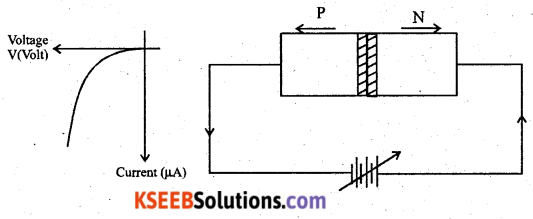Question 32.
Assuming the expression for the radius of electron orbit, obtain an expression for the total energy of the electron in the stationary orbit of the hydrogen atom.
Consider an electron of mass rn and charge -e revolving around the nucleus of an atom of atomic number Z in the nth orbit of radius ‘r’. Let v be the velocity of the electron. The electron possesses potential energy, because, it is in the electrostatic field of the nucleus. The electron also possesses kinetic energy by virtue of its motion.
The potential energy of the electron is given by,
Ep = (potential at a distance r from the nucleus) (-e)The kinetic energy of the electron is given by,
Ek = $$\frac {1}{2}$$ mv2 …….. (2)
From Bohr’s postulate,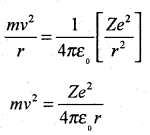Substituting this value of mv2 in equation (2)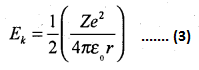The total energy of the electron revolving in the orbit is given by En = Ep + EkThe radius of nth permitted orbit of the electron is given by $$r=\frac{\varepsilon_{0} n^{2} h^{2}}{\pi m Z e^{2}}$$. Substituting this value of r in the equation,For the hydrogen atom, put Z = 1
Total energy of the electron in the nth orbit of hydrogen atom is $$E_{n}=\frac{-m e^{4}}{8 \varepsilon_{0}^{2} n^{2} h^{2}}$$
Note: The total energy of the electron in the first orbit is $$E_{1}=\frac{-m e^{4}}{8 \varepsilon_{0}^{2} h^{2}}=-13.6 \mathrm{eV}$$

VI. Answer any three of the following: (3 × 5 = 15)

Question 33.
The plates of a parallel plate capacitor have an area of 100 cm2 each and are separated by 3 mm. The capacitor is charged by connecting it to a 400 V supply.
(a) Calculate the electrostatic energy stored in the capacitor.
(b) If a dielectric of dielectric constant 2.5 is introduced between the plates of the capacitor, then find the electrostatic energy stored and also change in the energy stored.
Given A = 100 cm2 = 102 × 10-4 = 10-2 m2;
d = 3 mm = 3 × 10-3 m
V = 400 VQuestion 34.
In the given circuit diagram, calculate:
(i) The main current through the circuit and
(ii) Also current through 9 Ω resistor.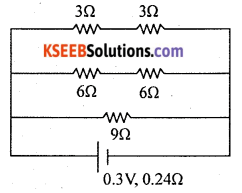Given R1 = 6 Ω
R2 = 12 Ω
R3 = 9 ΩP.d across internal resistance V = Ir = 1 × 0.24 = 0.24V
P.d across each resistors = 3 – 0.24 = 2.76 V
Current in 9 Ω = $$\frac{2.76}{9}$$ = 0.31 A ~ 0.3 A
Current in 9 Ω = 0.3 AQuestion 35.
A 20 Ω resistor, 1.5 H inductor, and 35 µF capacitors are connected in series with a 220 V, 50 Hz ac supply. Calculate the impedance of the circuit and also find the current through the circuit.Question 36.
The radii of curvature of two surfaces of a convex lens is 0.2m and 0.22m. Find the focal length of the lens if refractive index of the material of lens is 1.5. Also find the change in focal length, if it is immersed in water of refractive index 1.33.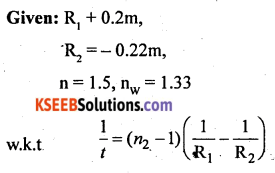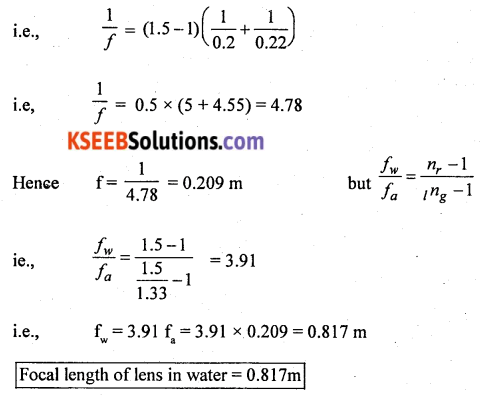The half-life of a radioactive sample $$_{38} \mathbf{S r}^{90}$$ is 28 years. Calculate the rate of disintegration of 15 mg of this isotope. Given Avogadro number = 6.023 × 1023.